# CLASS 11 MATHS CHAPTER 15-STATISTICS

### Exercise 15.1

Question1. Find the mean deviation about the mean for the data

4, 7, 8, 9, 10, 12, 13, 17

Solution :
The given data is

4, 7, 8, 9, 10, 12, 13, 17

Question2. Find the mean deviation about the mean for the data

38, 70, 48, 40, 42, 55, 63, 46, 54, 44

Solution :
The given data is

38, 70, 48, 40, 42, 55, 63, 46, 54, 44

Mean of the given data,

The deviations of the respective observations from the mean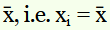are

–12, 20, –2, –10, –8, 5, 13, –4, 4, –6

The absolute values of the deviations, i.e.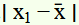, are

12, 20, 2, 10, 8, 5, 13, 4, 4, 6

The required mean deviation about the mean is

Question3. Find the mean deviation about the median for the data.

13, 17, 16, 14, 11, 13, 10, 16, 11, 18, 12, 17

Solution :
The given data is

13, 17, 16, 14, 11, 13, 10, 16, 11, 18, 12, 17

Here, the numbers of observations are 12, which is even.

Arranging the data in ascending order, we obtain

10, 11, 11, 12, 13, 13, 14, 16, 16, 17, 17, 18

The number of observations is 12

Then,

Median = ((12/2)th observation + ((12/2)+ 1)th observation)/2

(12/2)th observation = 6th = 13

(12/2)+ 1)th observation = 6 + 1

= 7th = 14

Median = (13 + 14)/2

= 27/2

= 13.5

So, the absolute values of the respective deviations from the median, i.e., |xi – M| are

3.5, 2.5, 2.5, 1.5, 0.5, 0.5, 0.5, 2.5, 2.5, 3.5, 3.5, 4.5

Mean Deviation,

= (1/12) × 28

= 2.33

Question4. Find the mean deviation about the median for the data

36, 72, 46, 42, 60, 45, 53, 46, 51, 49

Solution :

First we have to arrange the given observations into ascending order,

36, 42, 45, 46, 46, 49, 51, 53, 60, 72.

The number of observations is 10

Then,

Median = ((10/2)th observation + ((10/2)+ 1)th observation)/2

(10/2)th observation = 5th = 46

(10/2)+ 1)th observation = 5 + 1

= 6th = 49

Median = (46 + 49)/2

= 95

= 47.5

So, the absolute values of the respective deviations from the median, i.e., |xi – M| are

11.5, 5.5, 2.5, 1.5, 1.5, 1.5, 3.5, 5.5, 12.5, 24.5

Mean Deviation,

= (1/10) × 70

= 7

So, the mean deviation about the median for the given data is 7.

Question5. Find the mean deviation about the mean for the data.

Solution :

Question6. Find the mean deviation about the mean for the data

Solution :

Question7. Find the mean deviation about the median for the data.

Solution :
The given observations are already in ascending order.

Adding a column corresponding to cumulative frequencies of the given data, we obtain the following table.

Now, N = 26, which is even.

Median is the mean of the 13th and 14th observations. Both of these observations lie in the cumulative frequency 14, for which the corresponding observation is 7.

Then,

Median = (13th observation + 14th observation)/2

= (7 + 7)/2

= 14/2

= 7

So, the absolute values of the respective deviations from the median, i.e., |xi – M| are shown in the table.

.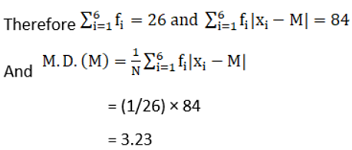Question8. Find the mean deviation about the median for the data

Solution :
The given observations are already in ascending order.

Adding a column corresponding to cumulative frequencies of the given data, we obtain the following table.

Here, N = 29, which is odd.

Now, N = 29, which is odd.

So 29/2 = 14.5

The cumulative frequency greater than 14.5 is 21, for which the corresponding observation is 30.

Then,

Median = (15th observation + 16th observation)/2

= (30 + 30)/2

= 60/2

= 30

So, the absolute values of the respective deviations from the median, i.e., |xi – M| are shown in the table.

Question9. Find the mean deviation about the mean for the data.

Solution :
The following table is formed.

Here,

Question10. Find the mean deviation about the mean for the data

Solution :
The following table is formed.

Question11. Find the mean deviation about median for the following data:

Solution :
The following table is formed.

The class interval containing Nth/2 or 25th item is 20-30

So, 20-30 is the median class.

Then,

Median = l + (((N/2) – c)/f) × h

Where, l = 20, c = 14, f = 14, h = 10 and n = 50

Median = 20 + (((25 – 14))/14) × 10

= 20 + 7.85

= 27.85

Question12. Calculate the mean deviation about median age for the age distribution of 100 persons given below:

Solution :
The given data is not continuous. Therefore, it has to be converted into continuous frequency distribution by subtracting 0.5 from the lower limit and adding 0.5 to the upper limit of each class interval.

The table is formed as follows.

The class interval containing Nth/2 or 50th item is 35.5 – 40.5

So, 35.5 – 40.5 is the median class.

Then,

Median = l + (((N/2) – c)/f) × h

Where, l = 35.5, c = 37, f = 26, h = 5 and N = 100

Median = 35.5 + (((50 – 37))/26) × 5

= 35.5 + 2.5

= 38

### Exercise 5.2

Question1. Find the mean and variance for the data 6, 7, 10, 12, 13, 4, 8, 12

Solution :
6, 7, 10, 12, 13, 4, 8, 12

Mean,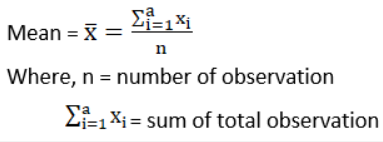x̅ = (6 + 7 + 10 + 12 + 13 + 4 + 8 + 12)/8

= 72/8

= 9

The following table is obtained.

Question2. Find the mean and variance for the first n natural numbers

Solution :

We know that Mean = Sum of all observations/Number of observations

∴Mean, x̅ = ((n(n + 1))2)/n

= (n + 1)/2

and also WKT Variance,

WKT, (a + b)(a – b) = a2 – b2

σ2 = (n2 – 1)/12

∴Mean = (n + 1)/2 and Variance = (n2 – 1)/12

Question3. Find the mean and variance for the first 10 multiples of 3

Solution :
The first 10 multiples of 3 are

3, 6, 9, 12, 15, 18, 21, 24, 27, 30

Here, number of observations, n = 10

The following table is obtained.

Then, Variance

= (1/10) × 742.5

= 74.25

∴Mean = 16.5 and Variance = 74.25

Question4. Find the mean and variance for the data

Solution :
The data is obtained in tabular form as follows.

Here, N = 40,

Question5. Find the mean and variance for the data

Solution :
The data is obtained in tabular form as follows.

Here, N = 22,

Question6. Find the mean and standard deviation using short-cut method.

Solution :
The data is obtained in tabular form as follows.

σ2 = (12/1002) [100(286) – 02]

= (1/10000) [28600 – 0]

= 28600/10000

= 2.86

Hence, standard deviation = σ = √2.886

= 1.691

∴ Mean = 64 and Standard Deviation = 1.691

Question7. Find the mean and variance for the following frequency distribution.

Solution :

Question8. Find the mean and variance for the following frequency distribution.

Solution :

Mean,

Question9. Find the mean, variance and standard deviation using short-cut method

Solution :

Mean,

Where, A = 92.5, h = 5

So, x̅ = 92.5 + ((6/60) × 5)

= 92.5 + ½

= 92.5 + 0.5

= 93

Then, Variance,

σ2 = (52/602) [60(254) – 62]

= (1/144) [15240 – 36]

= 15204/144

= 1267/12

= 105.583

Hence, standard deviation = σ = √105.583

= 10.275

∴ Mean = 93, variance = 105.583 and Standard Deviation = 10.275

Question10. The diameters of circles (in mm) drawn in a design are given below:

Solution :

Here, N = 100, h = 4

Let the assumed mean, A, be 42.5.

Mean,

Where, A = 42.5, h = 4

So, x̅ = 42.5 + (25/100) × 4

= 42.5 + 1

= 43.5

Then, Variance,

σ2 = (42/1002)[100(199) – 252]

= (1/625) [19900 – 625]

= 19275/625

= 771/25

= 30.84

Hence, standard deviation = σ = √30.84

= 5.553

∴ Mean = 43.5, variance = 30.84 and Standard Deviation = 5.553.

### Exercise 15.3

Question1. From the data given below state which group is more variable, A or B?

Solution :
Group A

Where A = 45,

and yi = (xi – A)/h

Here h = class size = 20 – 10

h = 10

So, x̅ = 45 + ((-6/150) × 10)

= 45 – 0.4

= 44.6

σ2 = (102/1502) [150(342) – (-6)2]

= (100/22500) [51,300 – 36]

= (100/22500) × 51264

= 227.84

Hence, standard deviation = σ = √227.84

= 15.09

∴ C.V for group A = (σ/ x̅) × 100

= (15.09/44.6) × 100

= 33.83

Group B

Where A = 45,

h = 10

So, x̅ = 45 + ((-6/150) × 10)

= 45 – 0.4

= 44.6

σ2 = (102/1502) [150(366) – (-6)2]

= (100/22500) [54,900 – 36]

= (100/22500) × 54,864

= 243.84

Hence, standard deviation = σ = √243.84

= 15.61

∴ C.V for group B = (σ/ x̅) × 100

= (15.61/44.6) × 100

= 35

By comparing C.V. of group A and group B.

C.V of Group B > C.V. of Group A

So, Group B is more variable.

Question2. From the prices of shares X and Y below, find out which is more stable in value:

Solution :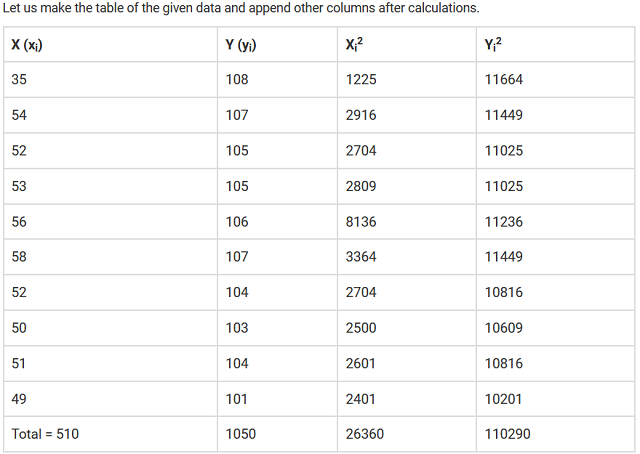We have to calculate Mean for x,

Mean x̅ = ∑xi/n

Where, n = number of terms

= 510/10

= 51

= (1/102)[(10 × 26360) – 5102]

= (1/100) (263600 – 260100)

= 3500/100

= 35

WKT Standard deviation = √variance

= √35

= 5.91

So, co-efficient of variation = (σ/ x̅) × 100

= (5.91/51) × 100

= 11.58

Now, we have to calculate Mean for y,

Mean ȳ = ∑yi/n

Where, n = number of terms

= 1050/10

= 105

= (1/102)[(10 × 110290) – 10502]

= (1/100) (1102900 – 1102500)

= 400/100

= 4

WKT Standard deviation = √variance

= √4

= 2

So, co-efficient of variation = (σ/ x̅) × 100

= (2/105) × 100

= 1.904

By comparing C.V. of X and Y.

C.V of X > C.V. of Y

So, Y is more stable than X.

Question3. An analysis of monthly wages paid to workers in two firms A and B, belonging to the same industry, gives the following results:

(i) Which firm A or B pays larger amount as monthly wages?

(ii) Which firm, A or B, shows greater variability in individual wages?

Solution :
(i) Monthly wages of firm A = Rs 5253

Number of wage earners in firm A = 586

∴Total amount paid = Rs 5253 × 586

Monthly wages of firm B = Rs 5253

Number of wage earners in firm B = 648

∴Total amount paid = Rs 5253 × 648

Thus, firm B pays the larger amount as monthly wages as the number of wage earners in firm B are more than the number of wage earners in firm A.

(ii) Variance of firm A = 100

We know that, standard deviation (σ)= √100

=10

Variance of firm B = 121

Then,

Standard deviation (σ)=√(121 )

=11

Hence the standard deviation is more in case of Firm B that means in firm B there is greater variability in individual wages.

Question4. The following is the record of goals scored by team A in a football session:

For the team B, mean number of goals scored per match was 2 with a standard deviation 1.25 goals. Find which team may be considered more consistent?

Solution :
The mean and the standard deviation of goals scored by team A are calculated as follows.

Question5. The sum and sum of squares corresponding to lengths x(in cm) and weight y(in gm) of 5- plant products are given below:

Which is more varying, the length or weight?

Solution :
Given:

### Miscellaneous Exercise

Question1. The mean and variance of eight observations are 9 and 9.25 respectively. If six of the observations are 6, 7, 10, 12, 12 and 13, find the remaining two observations.

Solution :
Let two required observations be x and y Then,

Question 2. The mean and variance of 7 observations are 8 and 16 respectively. If five of the observations are 2, 4, 10, 12, 14, find the remaining two observations.

Solution :
Let two required observations be x and y Then,

so, from eqn (i) ,we have

Question3. The mean and standard deviation of six observations are 8 and 4, respectively. If each observation is multiplied by 3, find the new mean and new standard deviation of the resulting observations.

Solution :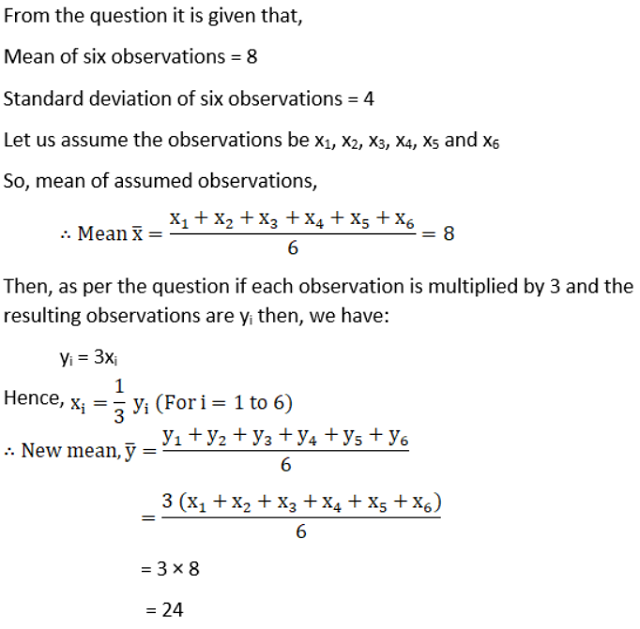Question4. Given that x̅ is the mean and σis the variance of n .observations x1, x2, …,xn ..Prove that the mean and variance of the observations ax1, ax2, ax3, …., axare ax̅ and a2σrespectively (a ≠ 0).

Solution :

From the question it is given that, n observations are x1, x2,…..xn

Mean of the n observation = x̅

Variance of the n observation = σ2

As we know that,

Question5. The mean and standard deviation of 20 observations are found to be 10 and 2 respectively. On rechecking, it was found that an observation 8 was incorrect. Calculate the correct mean and standard deviation in each of the following cases:

(i) if wrong item is omitted

(ii) if it is replaced by 12.

Solution :

(i) If wrong item is omitted,

From the question it is given that,

The number of observations i.e. n = 20

The incorrect mean = 20

The incorrect standard deviation = 2

(ii) If it is replaced by 12,

From the question it is given that,

The number of incorrect sum observations i.e. n = 200

The correct sum of observations n = 200 – 8 + 12

n = 204

Then, correct mean = correct sum/20

= 204/20

= 10.2

Question6. The mean and standard deviation of marks obtained by 50 students of a class in three subjects, Mathematics, Physics and Chemistry are given below:

Which of the three subjects shows the highest variability in marks and which shows the lowest?

Solution :

From the question it is given that,

Mean of Mathematics = 42

Standard deviation of Mathematics = 12

Mean of Physics = 32

Standard deviation of physics = 15

Mean of Chemistry = 40.9

Standard deviation of chemistry = 20

As we know that,

Therefore, Chemistry with highest C.V. shows highest variability and Mathematics with lowest C.V. shows lowest variability.

Question7. The mean and standard deviation of a group of 100 observations were found to be 20 and 3 respectively. Later on it was found that three observations were incorrect, which were recorded as 12, 21 and 18. Find the mean and standard deviation if the incorrect observations are omitted.

Solution :

From the question it is given that,

The total number of observations (n) = 100

Incorrect mean, (x̅) = 20

And, Incorrect standard deviation (σ) = 3

Get 30% off your first purchase!

X
error: Content is protected !!
Scroll to Top